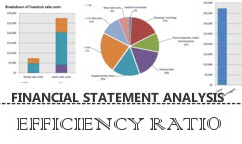Financial Statement Analysis - Efficiency Ratios

Efficiency ratios are ratios that come off the the Balance Sheet and the Income Statement and therefore incorporate one dynamic statement, the income statement and one static statement , the balance sheet. These ratios are important in measuring the efficiency of a company in either turning their inventory, sales, assets, accounts receivable or payable. It also ties into the ability of a company to meet both its short term and long term obligations. This is because if they do not get paid on time how will you get paid paid on time. You may have perhaps heard the excuse 'I will pay you when I get paid' or 'My customers have not paid me!'

##### 01 FIRST EFFICIENCY RATIO

DSO (Days Sales Outstanding): The Days Sales Outstanding ratio shows both the average time it takes to turn the receivables into cash and the age, in terms of days, of a company's accounts receivable. The ratio is regarded as a test of Efficiency for a company. The effectiveness with which it converts its receivables into cash. This ratio is of particular importance to credit and collection associates.

Best Possible DSO yields insight into delinquencies since it uses only the current portion of receivables. As a measurement, the closer the regular DSO is to the Best Possible DSO, the closer the receivables are to the optimal level.

Best Possible DSO requires three pieces of information for calculation:

•Current Receivables

•Total credit sales for the period analyzed

•The Number of days in the period analyzed

The Formula:

Best Possible DSO = Current Receivables/Total Credit Sales X Number of Days

The Formula:

Regular DSO = (Total Accounts Receivables/Total Credit Sales) x Number of Days in the period that is being analyzed

An example from our Balance sheet and Income Statement:

Total Accounts Receivables (from Balance Sheet) = \$97,456

Total Credit Sales (from Income Statement) = \$727,116

Number of days in the period = 1 year = 360 days ( some take this number as 365 days)

DSO = [ \$97,456 / \$727,116 ] x 360 = 48.25 days

The Interpretation:

Lumber & Building Supply Company takes approximately 48 days to convert its accounts receivables into cash. Compare this to their Terms of Net 30 days. This means at an average their customers take 18 days beyond terms to pay.

Review the Industry Norms and Ratios for this ratio to compare and see if they are above below or equal to the others in the same industry.

##### 02 SECOND EFFICIENCY RATIO

Inventory Turnover ratio: This ratio is obtained by dividing the 'Total Sales' of a company by its 'Total Inventory'. The ratio is regarded as a test of Efficiency and indicates the rapidity with which the company is able to move its merchandise.

The Formula:

Inventory Turnover Ratio = Net Sales / Inventory

It could also be calculated as:

Inventory Turnover Ratio = Cost of Goods Sold / Inventory

An example from our Balance sheet and Income Statement:

Net Sales = \$727,116 (from Income Statement)

Total Inventory = \$156,822 (from Balance sheet )

Inventory Turnover Ratio = \$727,116/ \$156,822

Inventory Turnover = 4.6 times

The Interpretation:

Lumber & Building Supply Company is able to rotate its inventory in sales 4.6 times in one fiscal year.

Review the Industry Norms and Ratios for this ratio to compare their efficiency and see if they are above, below or equal to the others in the same industry.

##### 03 THIRD EFFICIENCY RATIO

Accounts Payable to Sales (%): This ratio is obtained by dividing the 'Accounts Payables' of a company by its 'Annual Net Sales'. This ratio gives you an indication as to how much of their suppliers money does this company use in order to fund its Sales. Higher the ratio means that the company is using its suppliers as a source of cheap financing. The working capital of such companies could be funded by their suppliers..

The Formula:

Accounts Payable to Sales Ratio = [Accounts Payable / Net Sales ] x 100

An example from our Balance Sheet and Income Statement:

Accounts Payable = \$152,240 (from Balance sheet )

Net Sales = \$727,116 (from Income Statement)

Accounts Payable to Sales Ratio = [\$152,240 / \$727,116] x 100

Accounts Payable to Sales Ratio = 20.9%

The Interpretation:

21% of Lumber & Building Supply Company's Sales is being funded by its suppliers.

Review the Industry Norms and Ratios for this ratio to compare and see if they are above below or equal to the others in the same industry.

To use our online 'Accounts Payable to Sales Ratio Calculator' Click here.

Related Articles: Profitability Ratios

DMC Firewall is developed by Dean Marshall Consultancy Ltd